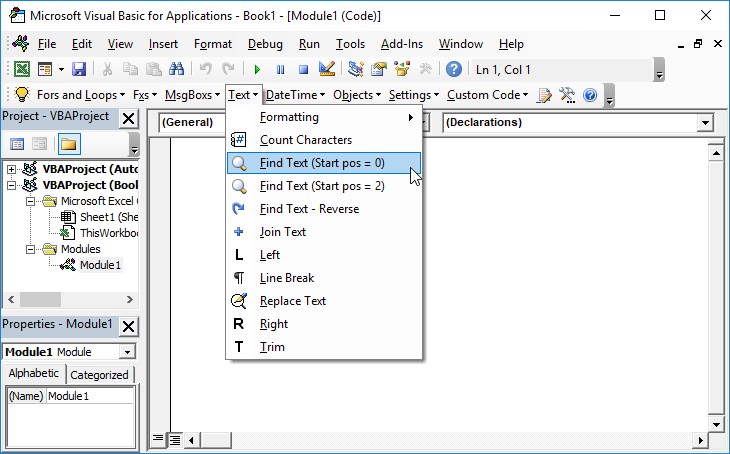# VBA – Find the nth Word in a String of Text

## Return nth Word in String of Text

The following function will find the nth word in a string:

``````Function Find_nth_word(Phrase As String, n As Integer) As String
Dim Current_Pos As Long
Dim Length_of_String As Integer
Dim Current_Word_No As Integer

Find_nth_word = ""
Current_Word_No = 1

Phrase = Trim(Phrase)

Length_of_String = Len(Phrase)

For Current_Pos = 1 To Length_of_String
If (Current_Word_No = n) Then
Find_nth_word = Find_nth_word & Mid(Phrase, Current_Pos, 1)
End If

If (Mid(Phrase, Current_Pos, 1) = " ") Then
Current_Word_No = Current_Word_No + 1
End If
Next Current_Pos

'Remove the rightmost space
Find_nth_word = Trim(Find_nth_word)

End Function``````

It needs two arguments – the phrase that is to be searched and the word number:

Find_nth_word(“Automateexcel ls the worlds favourite Excel site”,4)

Will return “world”.

A blank is returned if the number of words in the phrase is less than the word number specified.

So: Find_nth_word(“Automateexcel ls the worlds favourite Excel site”,12)
Will return blank as there are not 12 words in the phrase “Automateexcel ls the worlds favourite Excel site”.